# 石墨烯平面的卷曲与扭曲

• 2020-06-03 22:57:59

## 卷曲示例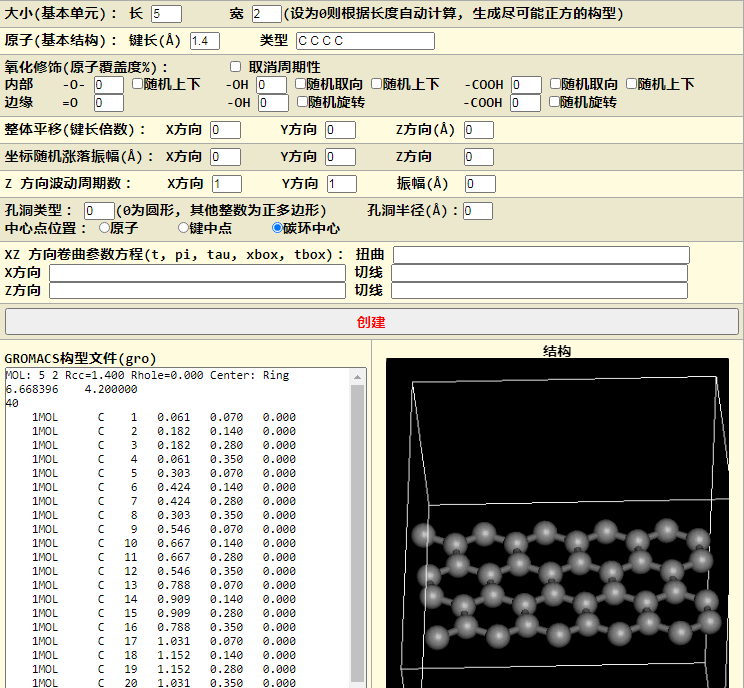$x=t \\ z=t$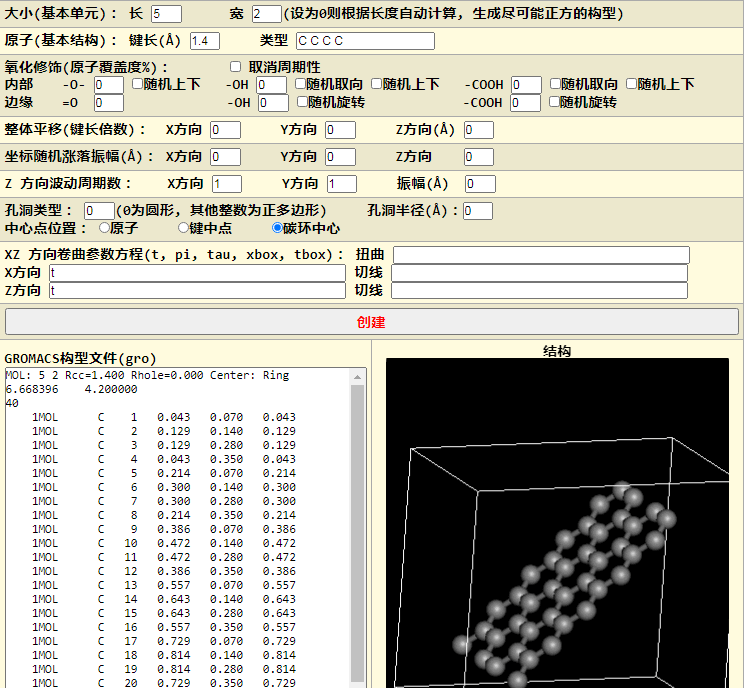$x=\cos(t) \\ z=\sin(t)$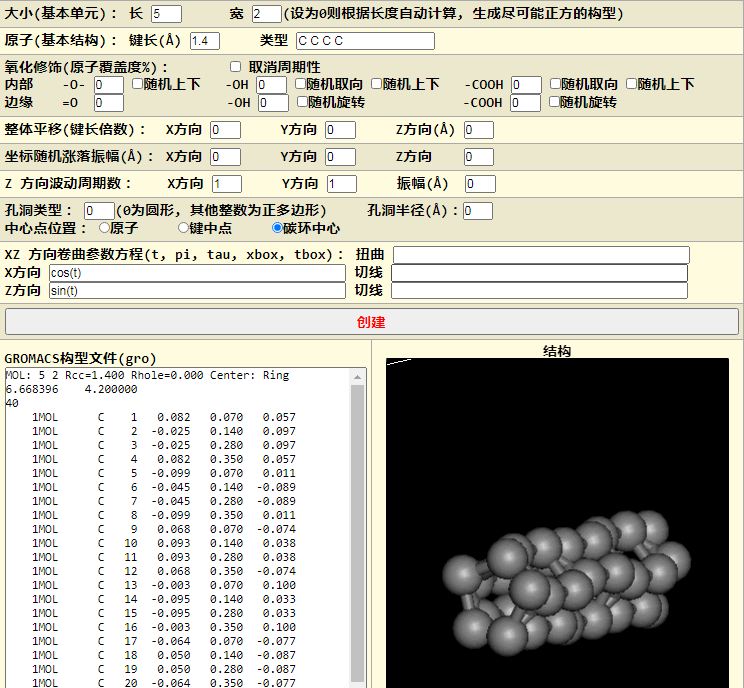$x=\text{xbox/pi}*\cos(t) \\ z=\text{xbox/pi}*\sin(t)$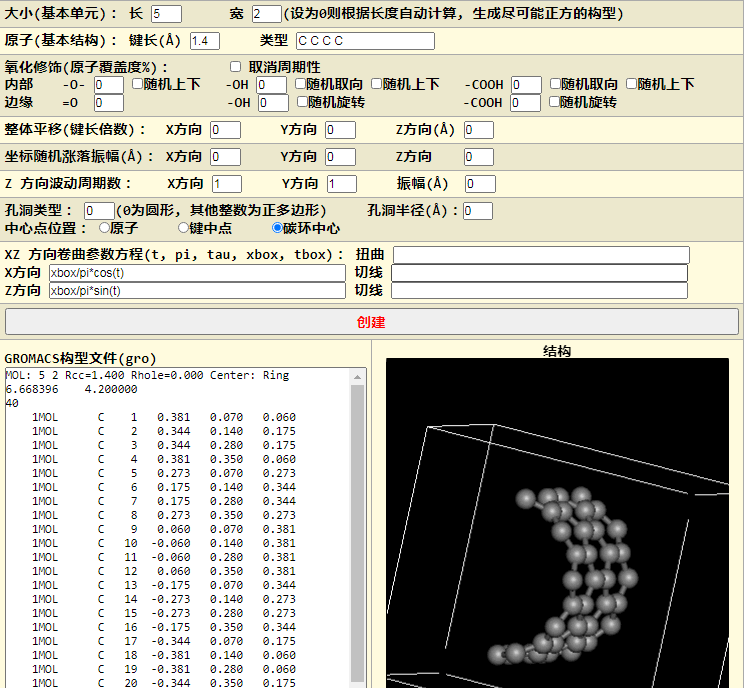$x=\text{xbox/tau}*\cos(t) \\ z=\text{xbox/tau}*\sin(t)$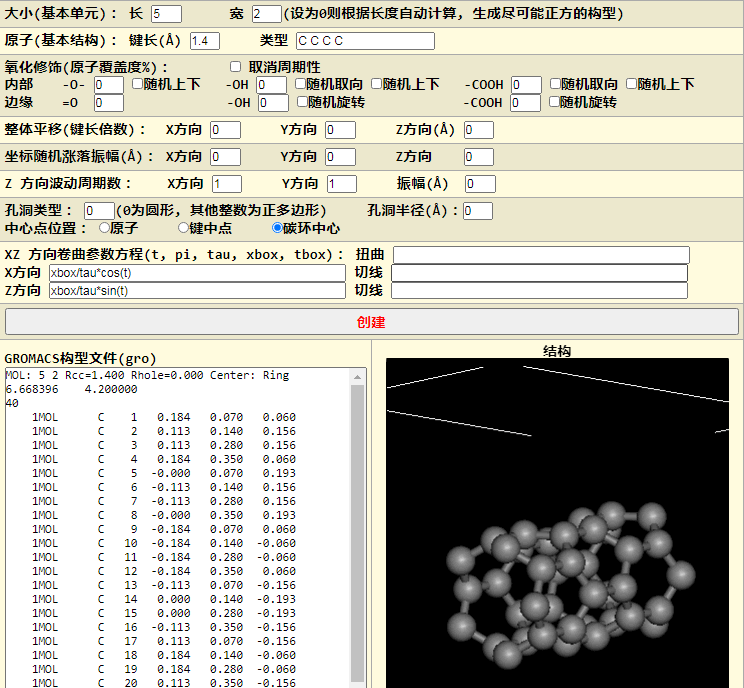$x=t*\cos(t) \\ z=t*\sin(t)$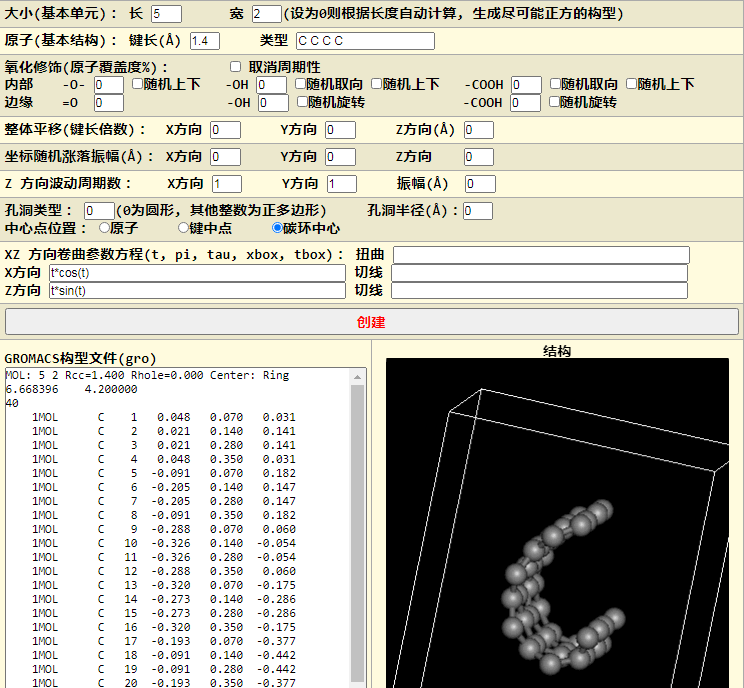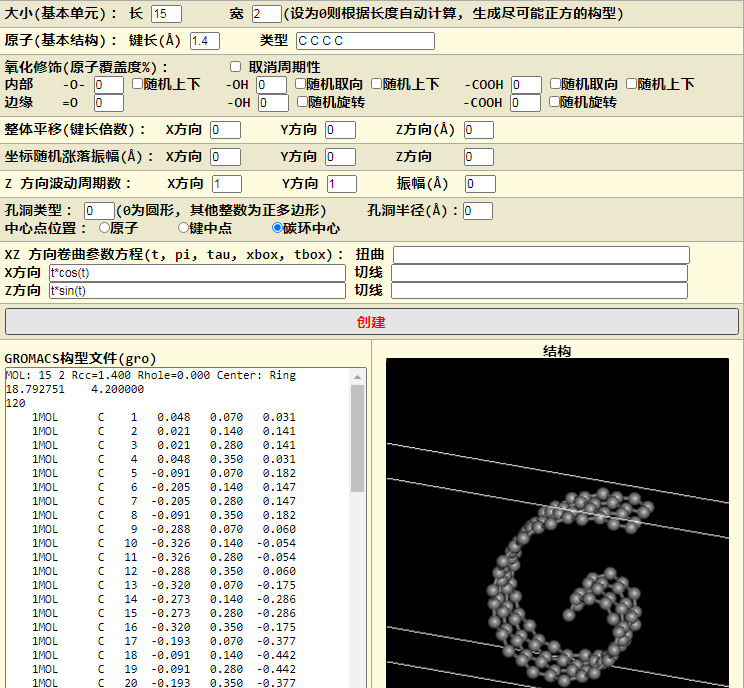$x=\cos(t)-.5*\cos(2*t) \\ z=\sin(t)-.5*\sin(2*t)$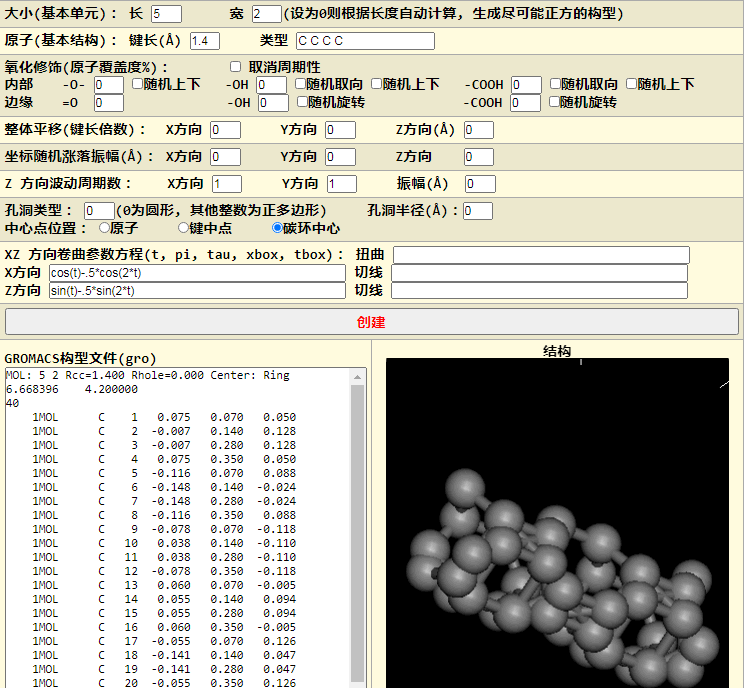$x=10*(\cos(t)-.5*\cos(2*t)) \\ z=10*(\sin(t)-.5*\sin(2*t))$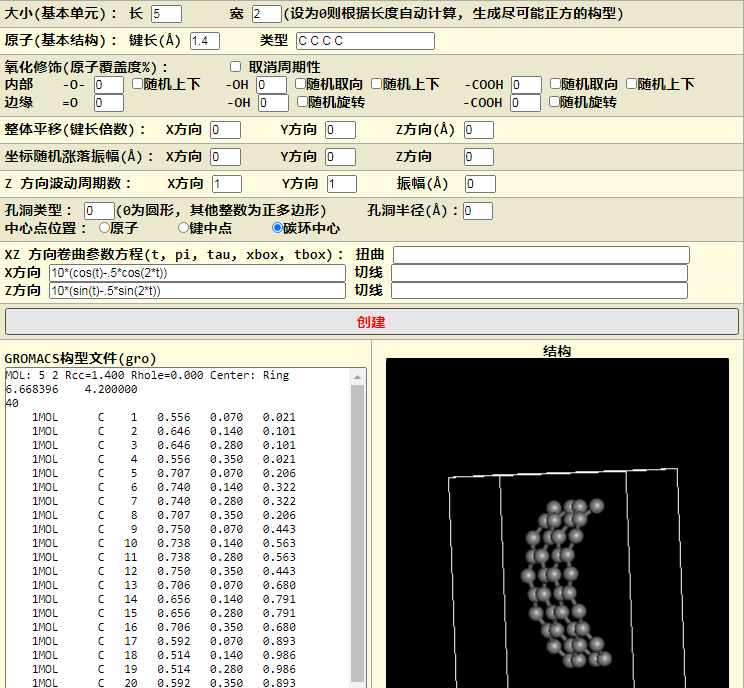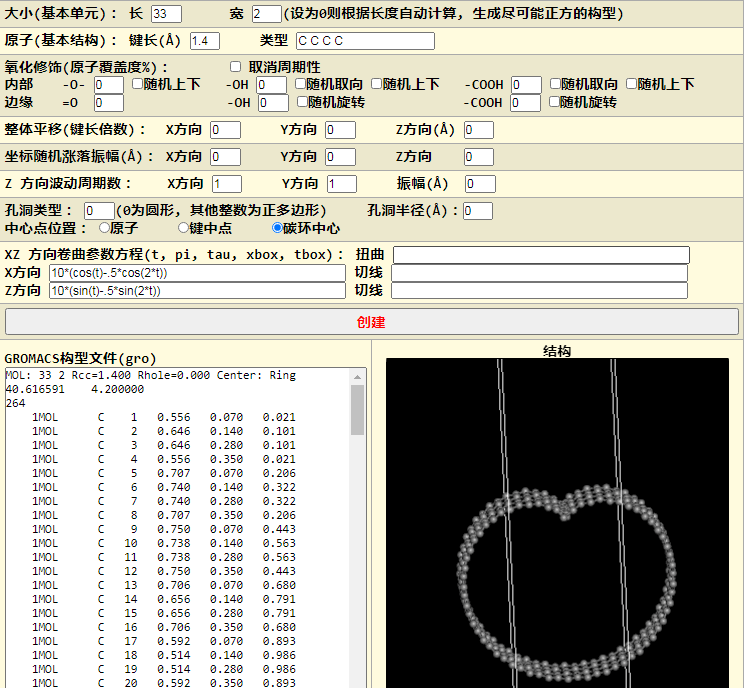$\alg x &=16*\text{pow}(\sin(t),3) \\ z &=13*\cos(t)-5*\cos(2*t)-2*\cos(3*t)-\cos(4*t) \ealg$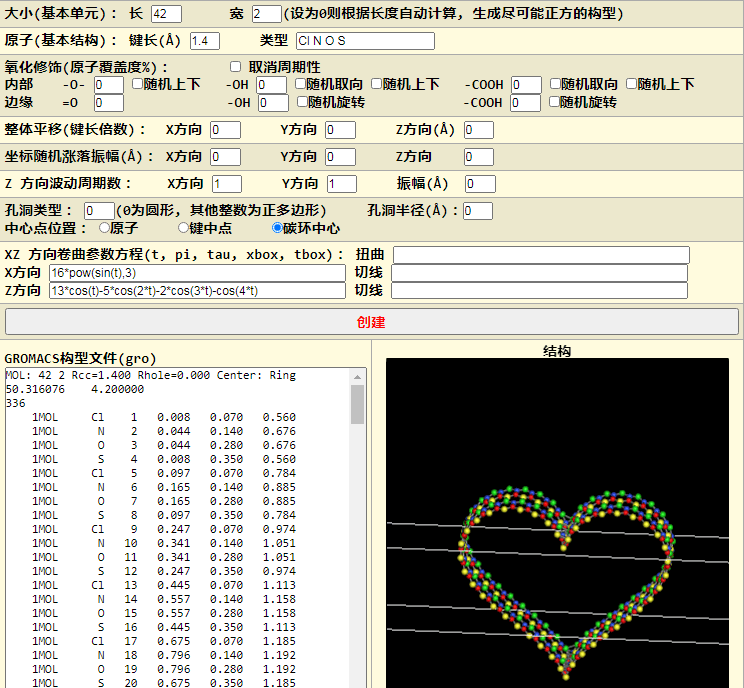## 扭曲示例

$t*\text{tau/tbox}$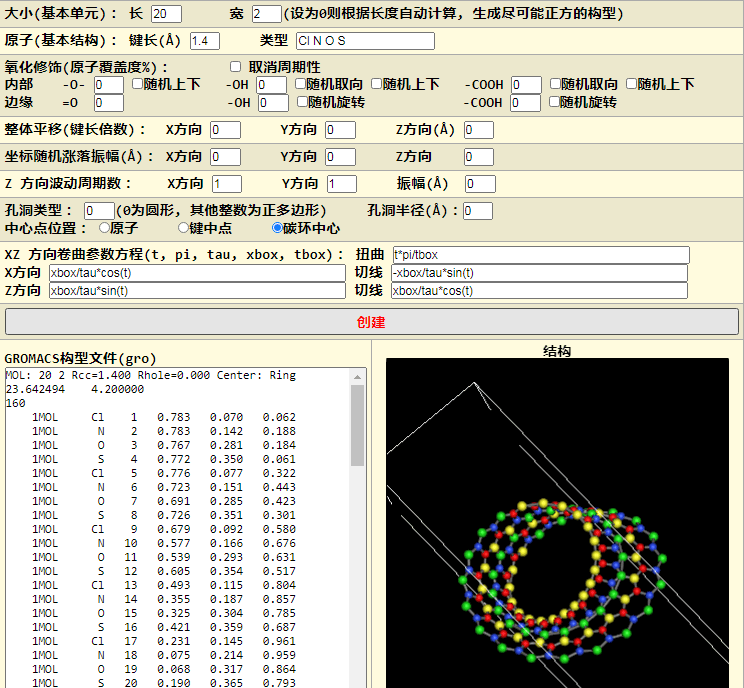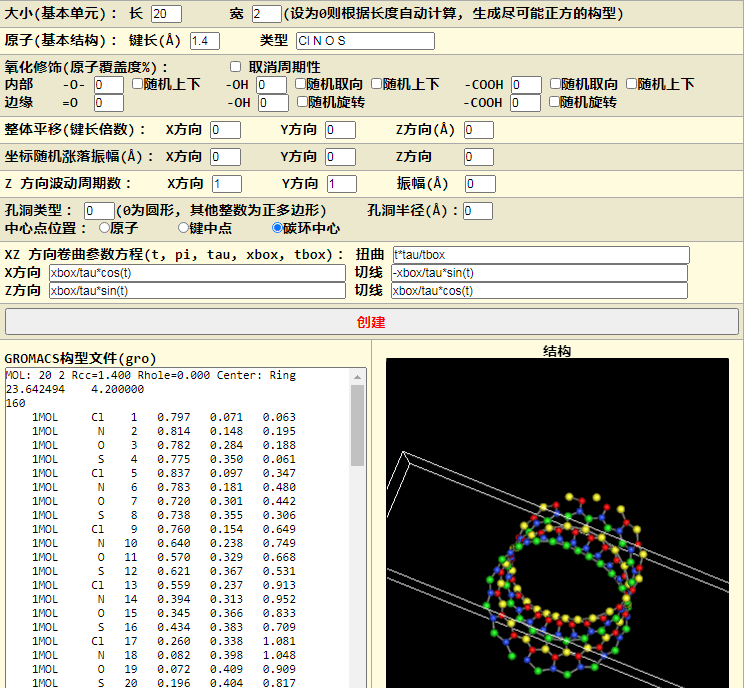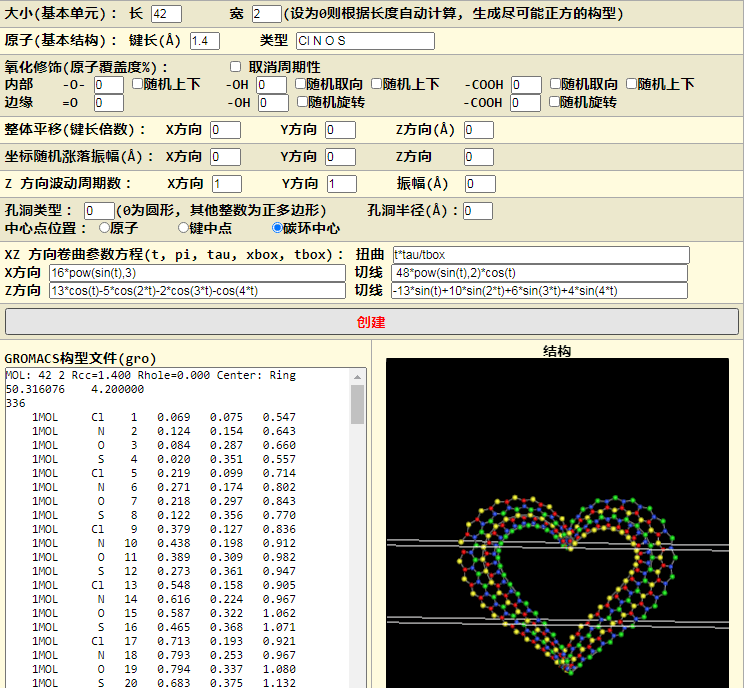## 遗留问题

1. 参数方程的系数问题. 对具有周期性的参数方程, 有没有简单的方法确定最合适的盒子长度, 使得所得构型正好对应一个周期? 对简单的圆环形状, 我们很容易确定. 对更复杂一些的, 如心脏线, 可以用试错法. 如何从理论上确定呢?
2. 我们只考虑了平面曲线, 能不能将其推广到空间曲线? 毕竟, 平面曲线只是空间曲线的一种特例而已. 答案是肯定的, 可能需要借助曲线的Frenet标架.
 随意赞赏微信支付宝
◆本文地址: , 转载请注明◆
◆评论问题: https://jerkwin.herokuapp.com/category/3/博客, 欢迎留言◆访问人次(2015年7月 9日起): | 最后更新: 2021-04-22 02:36:01 UTC | 版权所有 © 2008 - 2021 Jerkwin